Geometry

# Composite Figures Warmup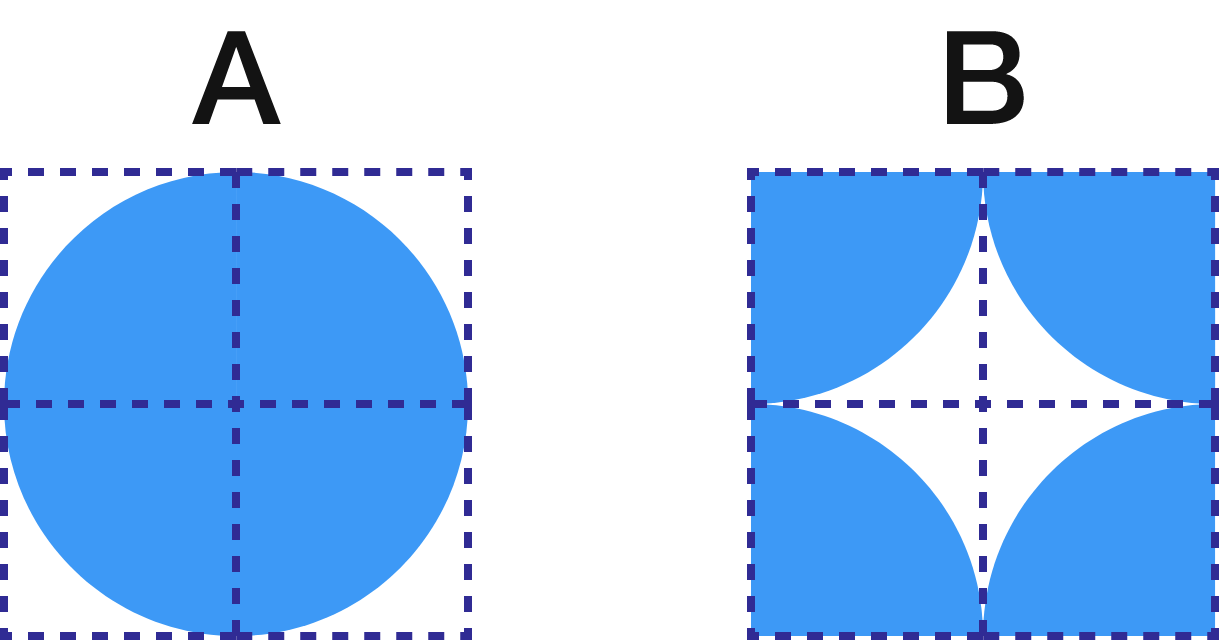In which figure is the shaded region greater?

(The squares are of equal size and the arcs are all of circles.)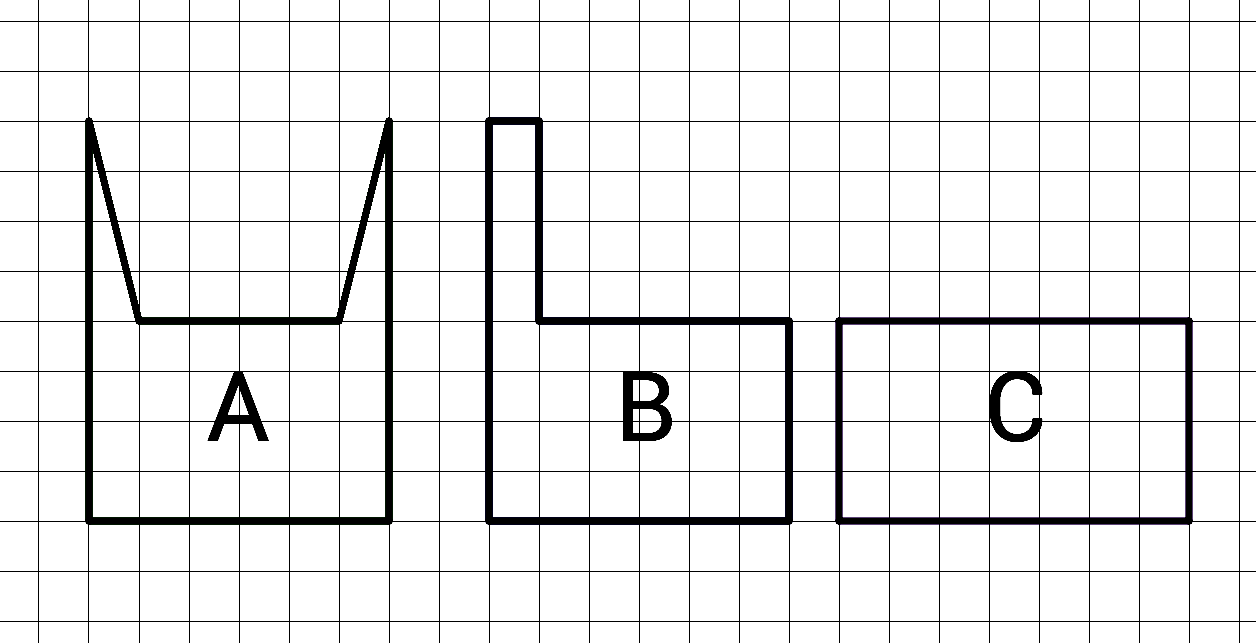Which figure has the greatest area?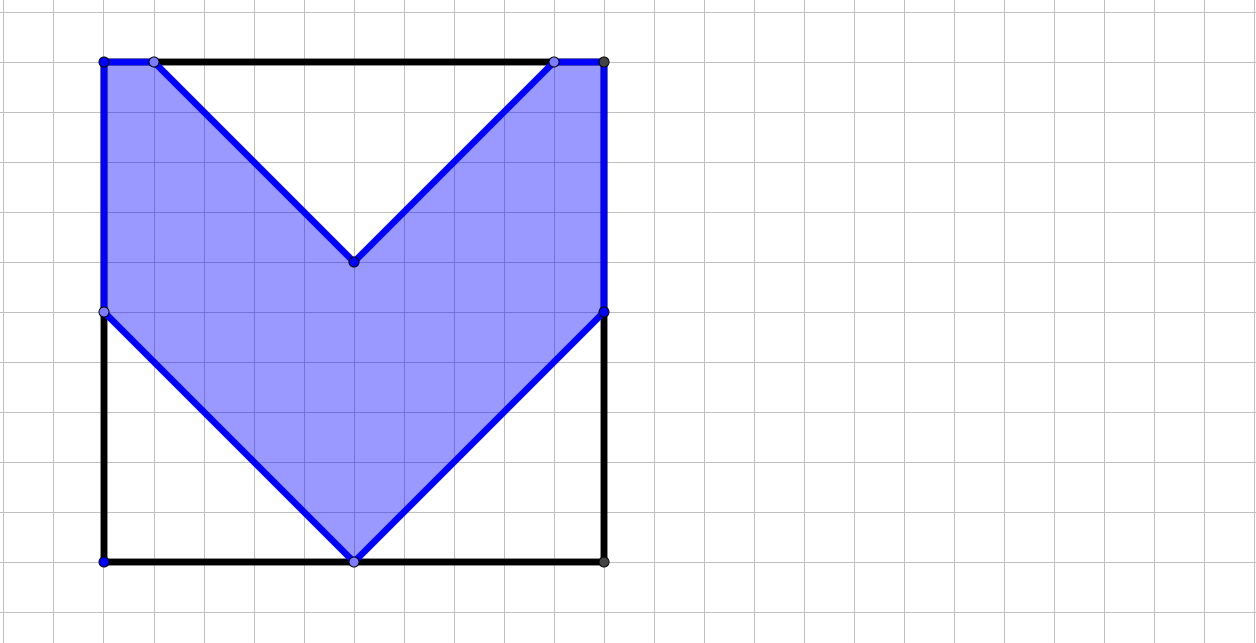If the square has side lengths of 10, what is the area of the blue $V?$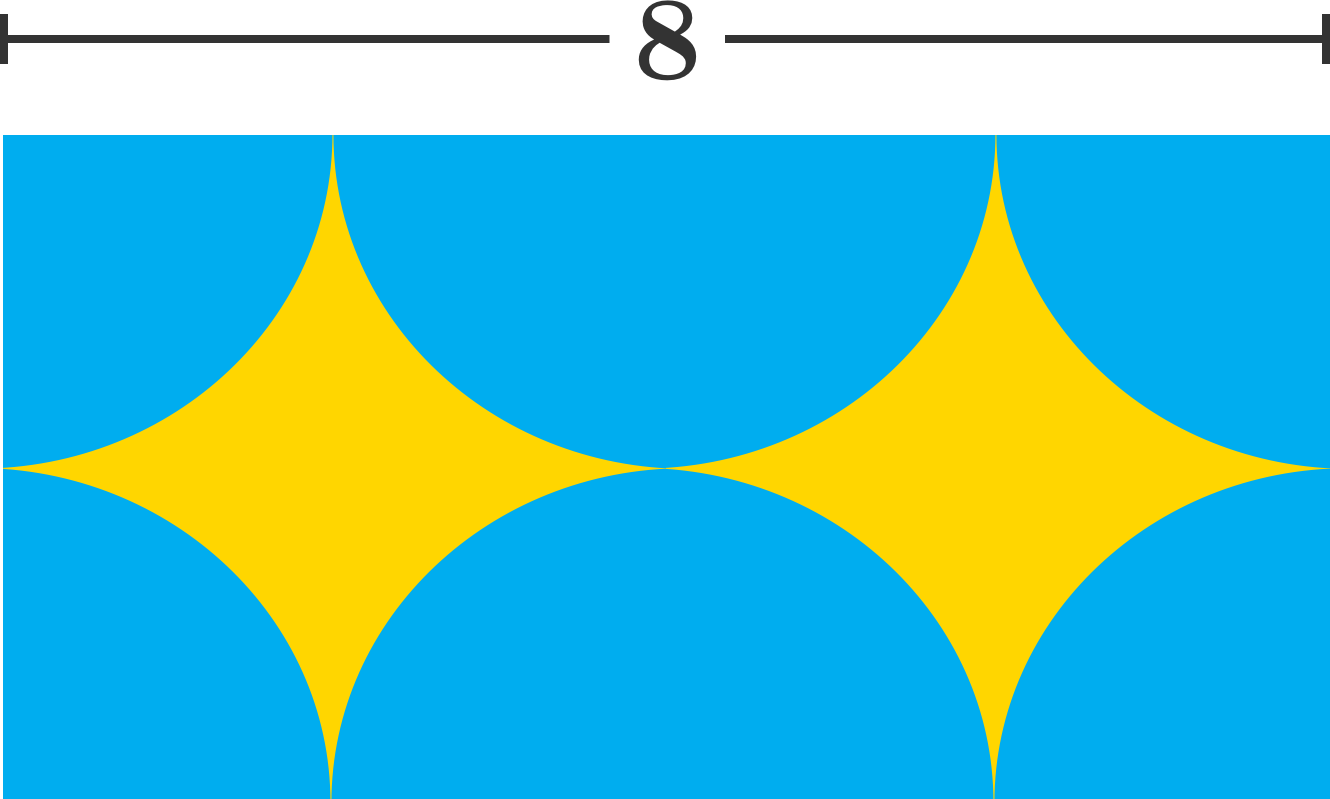If all circular pieces shown are semicircles and quarter circles, what is the area of the yellow region?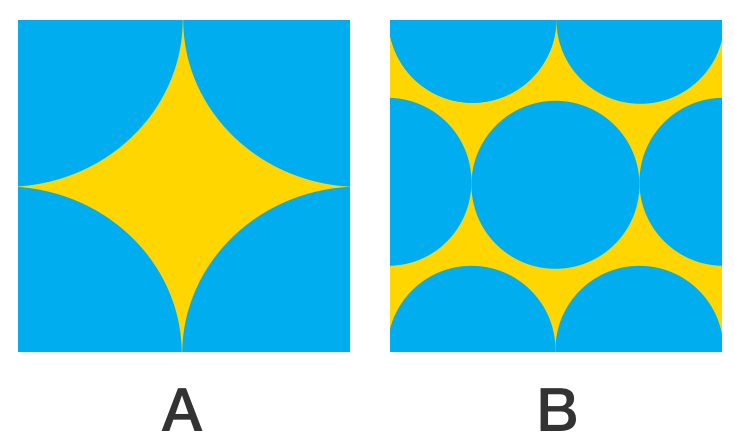Given that the squares are congruent, which figure has more dark blue area, $A$ or $B?$

Note: The left figure has four tangent quarter-circles, and the right figure has semicircles and a circle.

×